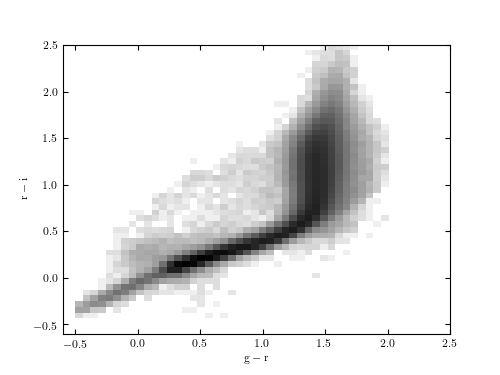# SDSS Stripe 82 Hess Diagram¶

Figure 1.10.

A Hess diagram of the r-i vs. g-r colors for the entire set of SDSS Stripe 82 standard stars. The pixels are colored with a logarithmic scaling; cf. figures 1.6 and 1.9.# Author: Jake VanderPlas
#   The figure produced by this code is published in the textbook
#   "Statistics, Data Mining, and Machine Learning in Astronomy" (2013)
#   To report a bug or issue, use the following forum:
import numpy as np
from matplotlib import pyplot as plt

from astroML.datasets import fetch_sdss_S82standards

#----------------------------------------------------------------------
# This function adjusts matplotlib settings for a uniform feel in the textbook.
# Note that with usetex=True, fonts are rendered with LaTeX.  This may
# result in an error if LaTeX is not installed on your system.  In that case,
# you can set usetex to False.
if "setup_text_plots" not in globals():
from astroML.plotting import setup_text_plots
setup_text_plots(fontsize=8, usetex=True)

#------------------------------------------------------------
# Fetch the stripe 82 data
data = fetch_sdss_S82standards()

g = data['mmu_g']
r = data['mmu_r']
i = data['mmu_i']

#------------------------------------------------------------
# Compute and plot the 2D histogram
H, xbins, ybins = np.histogram2d(g - r, r - i,
bins=(np.linspace(-0.5, 2.5, 50),
np.linspace(-0.5, 2.5, 50)))

# Create a black and white color map where bad data (NaNs) are white
cmap = plt.cm.binary

# Use the image display function imshow() to plot the result
fig, ax = plt.subplots(figsize=(5, 3.75))
H[H == 0] = 1  # prevent warnings in log10
ax.imshow(np.log10(H).T, origin='lower',
extent=[xbins, xbins[-1], ybins, ybins[-1]],
cmap=cmap, interpolation='nearest',
aspect='auto')

ax.set_xlabel(r'${\rm g - r}$')
ax.set_ylabel(r'${\rm r - i}$')

ax.set_xlim(-0.6, 2.5)
ax.set_ylim(-0.6, 2.5)

plt.show()